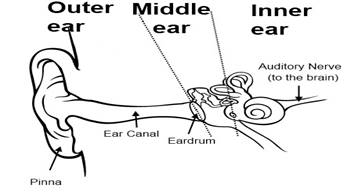### Sound - Solutions

CBSE class IX Science

NCERT Solutions
Chapter - 12
Sound

(Page No.162)

1. How does the sound produced by a vibrating object in a medium reach your ear?

Ans. As we speak, the particles of air near our mouth are pushed forward so they get compressed. Then they compress the other particles of air. As the compression proceeds the particles of air near our mouth expand again and thus rarefaction occurs. This process is repeated further and as a result sound wave propagates in the form of compressions and rarefactions to the listener’s ear.

(Page No.163)

1. Explain how sound is produced by your school bell.

Ans. When the peon strikes the school bell with a hammer, the particles of air near bell metal start vibrating and those vibrations produce sound.

2. Why are sound waves called mechanical waves?

Ans. Since sound waves need a medium for their propagation, therefore, we can say that sound waves are mechanical waves.

3. Suppose you and your friend are on the moon. Will you be able to hear any sound produced by your friend?

Ans. There is no air on moon hence there is no medium for sound propagation on the moon. As a result, I will not be able to hear any sound produced by my friend.

Page No.166)

1. Which wave property determines
(a) loudness,
(b) pitch?

Ans. (a) The amplitude of the wave determines the loudness of the sound.
(b) The frequency of the wave determines the pitch of the sound.

2. Guess which sound has a higher pitch: guitar or car horn?

Ans. The sound of Guitar has a higher pitch.

1. What are wavelength, frequency, time period and amplitude of a sound wave?

Ans. wavelength: For a sound wave, the combined length of a compression and an adjacent rarefaction is called its wavelength even the distance between centers of two consecutive compressions or two consecutive rarefactions is also equal to its wavelength.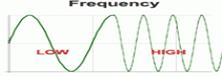frequency: The number of vibrations or oscillations per second is called frequency i.e. it is the number of complete waves or cycles produced in one second.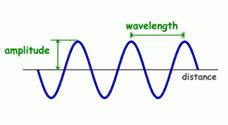time period: The time taken to complete one vibration/oscillation/complete wave is called time period. It is measured in seconds.

amplitude: It is the maximum displacement of the particles of the medium from their mean/original position at rest.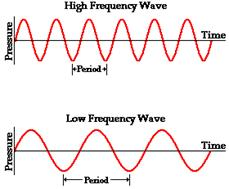2. How are the wavelength and frequency of a sound wave related to its speed?

Ans. From the equation: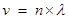where v = velocity/speed
n = frequency of wave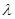= wavelength of wave

3. Calculate the wavelength of a sound wave whose frequency is 220 Hz and speed is 440 m/s in a given medium.

Ans. Since we know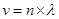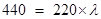= 440/220 = 2 m

4. A person is listening to a tone of 500 Hz sitting at a distance of 450 m from the source of the sound. What is the time interval between successive compressions from the source?

Ans. The time interval between successive compressions from the source
T = 1/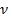= 1/500 = 0.002 second.

1. Distinguish between loudness and intensity of sound.

Ans.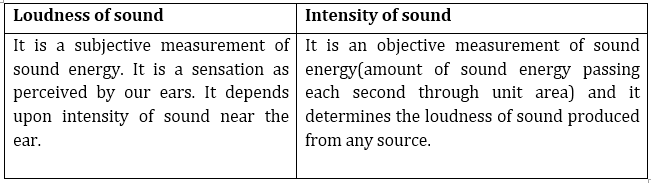(Page No.167)

1. In which of the three media, air, water or iron, does sound travel the fastest at a particular temperature?

Ans. The sound will travel the fastest in iron at a particular temperature.

(Page No.168)

1. An echo returned in 3 s. What is the distance of the reflecting surface from the source, given that the speed of sound is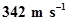?

Ans. Speed of sound = distance/time
therefore, distance travelled by sound during echo = speed x time = 342 x 3 = 1026 m
so the distance of reflecting surface = 1026/2 =513 m

(Page No.169)

1. Why are the ceilings of concert halls curved?

Ans. The ceilings of concert halls are curved because such architecture helps the sound to reach all the corners and places of the concert hall.

(Page No.170)

1. What is the audible range of the average human ear?

Ans. 20 Hz to 20,000Hz.

2. What is the range of frequencies associated with
(a) Infrasound?
(b) Ultrasound?

Ans. Infrasound = less than 20 Hz
Ultrasound = greater than 20 KHz

(Page No.172)

1. A submarine emits a sonar pulse, which returns from an underwater cliff in 1.02 s. If the speed of sound in salt water is 1531 m/s, how far away is the cliff?

Ans. Distance traveled by a sonar pulse = speed of sound in salt water x time
= 1531 x 1.02 = 1561.62 m
therefore, the distance of cliff from submarine = 1561.62/2 = 780.81 m

(Chapter – end)

1. What is sound and how is it produced?

Ans. A sound is a form of energy that produces a sensation of hearing in our ears. Sound gets produced when any object vibrates/oscillates.

2. Describe with the help of a diagram, how compressions and rarefactions are produced in the air near a source of the sound.

Ans.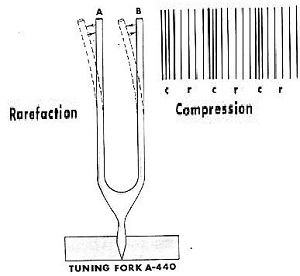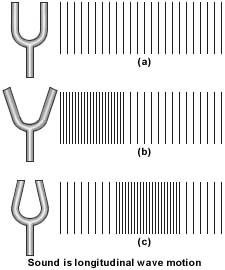A vibrating object producing a series of compressions (C) and rarefaction (R)
In these waves, the particles move back and forth parallel to the direction of propagation of the disturbance. Such waves are called longitudinal waves.
There is another kind of waves called transverse waves. In these waves, the particles oscillate up and down perpendicular to the propagation of the direction of the disturbance. Sound propagates in a medium as a series of compressions (C) and rarefactions (R). Compressions are the regions of high pressure and density where the particles are crowded and are represented by the upper portion of the curve called crest.Rarefactions are the regions of low pressure and density where the particles are spread out and are represented by the lower portion of the curve called trough.

3. Cite an experiment to show that sound needs a material medium for its propagation.

Ans. Take an electric bell and an airtight glass bell jar. The electric bell is suspended inside the airtight bell jar. The bell jar is connected to a vacuum pump If you press the switch you will be able to hear the bell. Now start the vacuum pump. When the air in the jar is pumped out gradually, the sound becomes fainter, although the same current is passing through the bell.
After some time when less air is left inside the bell jar you will hear a very feeble sound. Now if we evacuate the bell jar no sound is heard.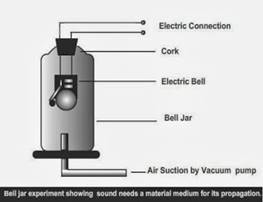Result: The above-mentioned activity shows that sound needs a medium to propagate.

4. Why is sound wave called a longitudinal wave?

Ans. The sound wave is called a longitudinal wave because particles of the medium through which the sound is transported vibrate parallel to the direction that the sound wave moves.

5. Which characteristic of the sound helps you to identify your friend by his voice while sitting with others in a dark room?

Ans. Pitch of the sound wave.

6. Flash and thunder are produced simultaneously. But thunder is heard a few seconds after the flash is seen, why?

Ans. Since the speed of thunder (sound) is much less (332 m/s) as compared to the speed of flash (light) which is about 3 x 108m/s therefore light travels faster than sound hence thunder is heard a few seconds after the flash is seen.

7. A person has a hearing range from 20 Hz to 20 kHz. What are the typical wavelengths of sound waves in air corresponding to these two frequencies? Take the speed of sound in air as 344 ms-1.

Ans. For 20 Hz sound waves, the wavelength would be= v/n = 344/20 = 17.2 m
For 20 kHz sound waves, the wavelength would be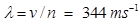/20000 Hz = 0.0172 m

8. Two children are at opposite ends of an aluminum rod. One strikes the end of the rod with a stone. Find the ratio of times taken by the sound wave in the air and in aluminum to reach the second child.

Ans. Since speed of sound in air = 344 m/s
and speed of sound in aluminum = 6420 m/s
we know that v = distance/time therefore time = d/v
time taken by sound wave in air/time taken by sound wave in aluminum
= d/344: d/6420 = 6420/344 = 18.66/1
the sound will take 18.66 times more time through the air than in aluminum in reaching another boy.

9. The frequency of a source of sound is 100 Hz. How many times does it vibrate in a minute?

Ans. The frequency of the source of sound being 100 Hz means the sound source vibrates 100 times in one second.
therefore vibrations made by sound source in 1 min (60 sec) = 100 x 60 = 6000

10. Does sound follow the same laws of reflection as light does? Explain.

Ans. Yes. Sound follows the same laws of reflection as light does. We can say that because here the directions in which the sound is incident and is reflected make equal angles with the normal to the reflecting surface at the point of incidence, and the three are in the same plane.

11. When a sound is reflected from a distant object, an echo is produced. Let the distance between the reflecting surface and the source of sound production remains the same. Do you hear echo sound on a hotter day?

Ans. As the sensation of sound persists in our brain for about 0.1 s.To hear a distinct echo the time interval between the original sound and the reflected one must be at least 0.1s. There for the total distance covered by the sound from the point of generation to the reflecting surface and back should be at least (344m/s) x 0.1s = 34.4 m. Thus, for hearing distinct echoes, the minimum distance of the obstacle from the source of sound must be half of this distance, that is, 17.2 m. The speed of sound will increase with an increase in temperature. Therefore, on a hotter day speed of sound will be greater hence reflected sound will reach us before 0.1 seconds.As a result, there will be no distinct echo will be heard by us.

12. Give two practical applications of reflection of sound waves.

Ans. Two practical applications of reflection of sound waves
i. Megaphones or loudhailers, horns, musical instruments such as trumpets and shehanais, are all designed to send sound in a particular direction without spreading it in all directions.ii.The stethoscope is a medical instrument used for listening to sounds produced within the body, chiefly in the heart or lungs. In stethoscopes, the sound of the patient’s heartbeat reaches the doctor’s ears by multiple reflections of sound.

13. A stone is dropped from the top of a tower 500 m high into a pond of water at the base of the tower. When is the splash heard at the top? Given, g = 10ms-2 and speed of sound = 340 ms-1.

Ans.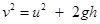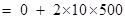= 10000
v =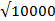= 100 m/s
we also know that v = u + gt = 0 + 10t
100 = 10t or, Time taken by stone to reach the pond surface (t) = 100/10 = 10 sec
therefore, time taken by sound to reach the top from pond surface = d/v = 500/340
= 1.47 sec
so the total time taken for splash being heard at the top = 10 + 1.47 = 11.47 s

14. A sound waves travels at a speed of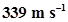. If its wavelength is 1.5 cm, what is the frequency of the wave? Will it be audible?

Ans. Since we know that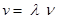339 = 0.015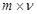ν = 339/0.015 = 22600 Hz
Since the resulting frequency is beyond the audible range of human beings (20Hz to 20kHz) therefore sound will not be audible to human ears.

15. What is reverberation? How can it be reduced?

Ans. The repeated reflection of sound due to which sound persists for a long time is called reverberation.
To reduce reverberation, the roof and walls of the auditorium are generally covered with sound-absorbent materials like compressed fibreboard, rough plaster or draperies. The seat materials are also selected on the basis of their sound absorbing properties.

16. What is loudness of sound? What factors does it depend on?

Ans. Loudness is a measure of the response of the ear to the sound. Even when two sounds are of equal intensity, we may hear one as louder than the other simply because our ear detects it better.
The loudness of a sound depends upon the amplitude of those sound waves. Higher is the amplitude of vibrating air particles louder will be the sound.

17. Explain how bats use ultrasound to catch a prey.

Ans. Bats search out prey and fly in dark night by emitting and detecting reflections of ultrasonic waves. The high-pitched ultrasonic squeaks of the bat are reflected from the obstacles or prey and returned to bat’s ear. The nature of reflections tells the bat where the obstacle or prey is and what it is like.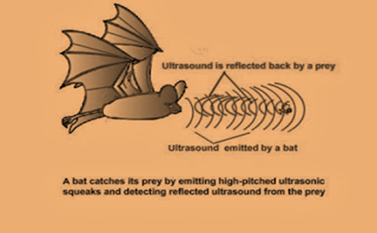18. How is ultrasound used for cleaning?

Ans. Ultrasound is generally used to clean parts located in hard-to-reach places, for example, spiral tube, odd shaped parts, electronic components etc. Objects to be cleaned are placed in a cleaning solution and ultrasonic waves are sent into the solution. Due to the high frequency, the particles of dust, grease, and dirt get detached and drop out. The objects thus get thoroughly cleaned.

19. Explain the working and application of a sonar.

Ans. Sonar is a device that uses ultrasonic waves to measure the distance, direction, and speed of underwater objects.
Sonar consists of a transmitter and a detector and is installed in a boat or a ship. The transmitter produces and transmits ultrasonic waves. These waves travel through water and after striking the object on the seabed, get reflected back and are sensed by the detector. The detector converts the ultrasonic waves into electrical signals which are appropriately interpreted. The distance of the object that reflected the sound wave can be calculated by knowing the speed of sound in water and the time interval between transmission and reception of the ultrasound. Let the time interval between transmission and reception of ultrasound signal be and the speed of sound through seawater be v. The total distance, 2traveled by the ultrasound is then, 2d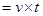or  $d=\frac{v×t}{2}$
The above method is called echo-ranging. The sonar technique is used to determine the depth of the sea and to locate underwater hills, valleys, submarine, icebergs, sunken ship etc.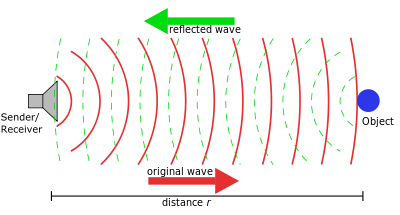20. A sonar device on a submarine sends out a signal and receives an echo 5 s later. Calculate the speed of sound in water if the distance of the object from the submarine is 3625 m.

Ans. Distance of object from submarine = 3625 m

therefore, distance travelled by sonar waves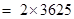= 7250 m

since, speed = distance/time= 7250/5 = 1450 m/s

21. Explain how defects in a metal block can be detected using ultrasound.

Ans. Ultrasounds can be used to detect cracks and flaws in metal blocks. Metallic components are generally used in the construction of big structures like buildings, bridges, machines and also scientific equipment.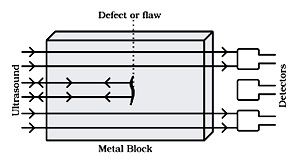The cracks or holes inside the metal blocks, which are invisible from outside reduces the strength of the structure. Ultrasonic waves are allowed to pass through the metal block and detectors are used to detect the transmitted waves. If there is even a small defect, the ultrasound gets reflected back indicating the presence of the flaw or defect

22. Explain how the human ear works.

Ans. The outer ear is called ‘pinna’. It collects the sound from the surroundings. The collected sound passes through the auditory canal. At the end of the auditory canal, there is a thin membrane called the eardrum or tympanic membrane. When a compression of the medium reaches the eardrum the pressure on the outside of the membrane increases and forces the eardrum inward. Similarly, the eardrum moves outward when a rarefaction reaches it. In this way, the eardrum vibrates. The vibrations are amplified several times by three bones (the hammer, anvil, and stirrup) in the middle ear. The middle ear transmits the amplified pressure variations received from the sound wave to the inner ear. In the inner ear, the pressure variations are turned into electrical signals by the cochlea. These electrical signals are sent to the brain via the auditory nerve, and the brain interprets them as sound.